Theory and Modern Applications

# Permanence and existence of positive periodic solution for a multi-species cooperation system with continuous time delays, feedback control, and periodic external source

## Abstract

In this paper, sufficient conditions which guarantee the existence of positive periodic solutions for a multi-species cooperation system are obtained. The permanence of solutions is studied. The proof is based on Schauder’s fixed-point theorem. Also, we give a illustrative example in order to indicate the validity of the assumptions.

MSC:54H99.

## 1 Introduction

May suggested the following system equations  as the mathematical modeling of the pair of mutualist:

$\begin{array}{c}\stackrel{˙}{x}={\rho }_{1}x\left[1-\frac{x}{{a}_{1}+{b}_{1}y}-{c}_{1}x\right],\hfill \\ \stackrel{˙}{y}={\rho }_{2}y\left[1-\frac{y}{{a}_{2}+{b}_{2}x}-{c}_{2}y\right],\hfill \end{array}$

where $x\left(t\right)$, $y\left(t\right)$ are densities of the species X, Y at time t, ${\rho }_{i}$, ${a}_{i}$, ${b}_{i}$, ${c}_{i}$, $i=1,2$ are positive constants. Subsequently, the nonautonomous version was argued by Cui and Chen . Cui in  proposed the following generalization for the N-species cooperation system with continuous time delays:

(1.1)

where ${\int }_{-\tau }^{0}{V}_{j}\left(\theta \right)\phantom{\rule{0.2em}{0ex}}d\theta =1$, $j=1,2,\dots ,N$.

On the other hand, in the more realistic situation, the cooperation systems or ecosystems are continuously perturbed via unpredictable forces. These perturbations are generally results of the change in the system’s parameters. In the language of the control theory, these perturbation functions may be regarded as control variables, and consequently, one should ask the question that whether or not an ecosystem can withstand those unpredictable perturbations which persist for a finite periodic time. During the last decade, many scholars did works on the feedback control ecosystems. Some results can be found in  and the references therein. Chen, Lio, and Huang  studied the dynamical behavior of the following non-autonomous N-species cooperation system with continuous time delay and feedback control: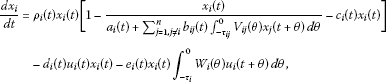(1.2)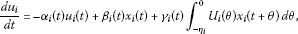(1.3)

where ${x}_{i}$ and ${u}_{i}$ are the density of i th cooperation species and control variable, respectively. ${a}_{i}$, ${b}_{ij}$, ${c}_{i}$, ${d}_{i}$, ${\rho }_{i}$, ${\alpha }_{i}$, ${\beta }_{i}$, and ${\gamma }_{i}$, are all continuous real-valued functions which are bounded above and below by positive constants. Also,

${\int }_{-{\tau }_{ij}}^{0}{V}_{i}\left(\theta \right)\phantom{\rule{0.2em}{0ex}}d\theta ={\int }_{-{\tau }_{i}}^{0}{W}_{i}\left(\theta \right)\phantom{\rule{0.2em}{0ex}}d\theta ={\int }_{-{\eta }_{i}}^{0}{U}_{i}\left(\theta \right)\phantom{\rule{0.2em}{0ex}}d\theta =1,\phantom{\rule{1em}{0ex}}i,j=1,2,\dots ,N.$

Very recently, Chen and Xie  obtained a set of sufficient conditions for permanence of the system above. The study was based on a new integral inequality and the results showed that the feedback control variables have no influence on the permanence of the system. In the present paper, we study the sufficient condition for existence and permanence of the positive periodic solutions of generalized version of the N-species cooperation system (1.2), (1.3) while for each i, time variation of the i th species is affected by external periodic source ${S}_{i}\left(t\right)$, i.e.,(1.4)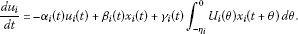(1.5)

Our key tool is the following fixed-point theorem for a proper compact integral operator on the convex subset of infinite dimension Banach space which is originally due to Schauder .

Theorem 1.1 (Schauder)

Let X be a Banach space and Λ be a closed, bounded, and convex subset of X. If $\mathrm{\Gamma }:\mathrm{\Lambda }↦\mathrm{\Lambda }$ is a compact operator, then Γ has at least one fixed point on Λ.

Besides, we also invoke the following weak version of Arzela-Ascoli theorem .

Theorem 1.2 (Arzela-Ascoli)

Let $\left\{{\xi }_{n}\left(t\right)\right\}$ be a sequence of real functions on $\left[0,T\right]$ which is uniformly bounded and equicontinuous. Then $\left\{{\xi }_{n}\left(t\right)\right\}$ has a uniformly convergent subsequence.

Also, we set,

and for $\xi \in {\mathcal{C}}_{T}$ we define $\parallel \xi \parallel ={sup}_{t\in \left[0,T\right]}|\xi \left(t\right)|$,

$\mathcal{B}={\mathcal{C}}_{T}^{N}=\left\{\mathrm{\Psi }=\left({\xi }_{1},{\xi }_{2},\dots ,{\xi }_{N}\right):{\xi }_{i}\in {\mathcal{C}}_{T},i=1,2,\dots ,N\right\},$

and for $\mathrm{\Psi }\in \mathcal{B}$ we define $|\mathrm{\Psi }\left(t\right)|={\sum }_{i=1}^{N}|{\xi }_{i}\left(t\right)|$ and ${\parallel \mathrm{\Psi }\parallel }_{\mathcal{B}}={\sum }_{i=1}^{N}\parallel {\xi }_{i}\parallel$.

Clearly, $\left({\mathcal{C}}_{T},\parallel \cdot \parallel \right)$ and $\left(\mathcal{B},{\parallel \cdot \parallel }_{\mathcal{B}}\right)$ are Banach spaces.

$\begin{array}{c}{\lambda }_{i}=exp\left({\int }_{0}^{T}{\rho }_{i}\left(t\right)\phantom{\rule{0.2em}{0ex}}dt\right),\phantom{\rule{2em}{0ex}}{\kappa }_{i}=exp\left({\int }_{0}^{T}{\alpha }_{i}\left(t\right)\phantom{\rule{0.2em}{0ex}}dt\right),\hfill \\ {A}_{i}={\int }_{0}^{T}\left\{{\beta }_{i}\left(t\right)+{\gamma }_{i}\left(t\right)\right\}\phantom{\rule{0.2em}{0ex}}dt,\phantom{\rule{2em}{0ex}}{B}_{i}={\int }_{0}^{T}\left\{{d}_{i}\left(t\right)+{e}_{i}\left(t\right)\right\}\phantom{\rule{0.2em}{0ex}}dt,\hfill \\ {J}_{i}\left(Q\right)={\int }_{0}^{T}{\rho }_{i}\left(s\right)\left[\frac{1}{{a}_{i}\left(s\right)+Q{\sum }_{j=1,j\ne i}^{n}{b}_{ij}\left(s\right)}+{c}_{i}\left(s\right)\right]\phantom{\rule{0.2em}{0ex}}ds,\phantom{\rule{1em}{0ex}}Q\in \mathbb{R},\hfill \\ {I}_{i}\left(Q\right)=\frac{1}{{inf}_{t\in \left[0,T\right]}\left[{a}_{i}\left(t\right)+Q{\sum }_{j=1,j\ne i}^{n}{b}_{ij}\left(t\right)\right]}+\parallel {c}_{i}\parallel .\hfill \end{array}$

Throughout this paper, we assume that

(${H}_{1}$) ${a}_{i}$, ${b}_{ij}$, ${c}_{i}$, ${d}_{i}$, ${e}_{i}$, ${\rho }_{i}$, ${\alpha }_{i}$, ${\beta }_{i}$, and ${\gamma }_{i}$, $i,j=1,2,\dots ,N$ are all positive continuous real-valued functions.

(${H}_{2}$) ${\int }_{-{\tau }_{ij}}^{0}{V}_{i}\left(\theta \right)\phantom{\rule{0.2em}{0ex}}d\theta ={\int }_{-{\tau }_{i}}^{0}{W}_{i}\left(\theta \right)\phantom{\rule{0.2em}{0ex}}d\theta ={\int }_{-{\eta }_{i}}^{0}{U}_{i}\left(\theta \right)\phantom{\rule{0.2em}{0ex}}d\theta =1$, $i,j=1,2,\dots ,N$.

The following section is arranged based on two main steps: In step 1, we obtain sufficient conditions with guarantee the existence of periodic solutions of each equation of system (1.4)-(1.5) for each $1\le i\le N$. In the proof of existence, we will use the method of Green’s functions according to Mokhtarzadeh et al. . In step 2, we follow Chen  to construct a bounded, closed, and convex set in a product space and apply Schauder’s fixed-point theorem.

## 2 Main results

In this section, we shall study the existence of periodic solutions of the multi-species system (1.4)-(1.5). To do this, we transform this system of couple equations into one integral equation. For each $1\le i\le N$, we introduce the following integral operator ${\mathrm{\Gamma }}_{i}$ on the Banach space $\left({\mathcal{C}}_{T},\parallel \cdot \parallel \right)$,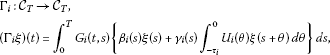(2.1)

The kernel of the integral operator (2.1) is in fact the Green’s function of Eq. (1.2) and is given by

${G}_{i}\left(t,s\right)=\left\{\begin{array}{cc}\frac{exp\left({\int }_{0}^{T}{\alpha }_{i}\left(\theta \right)\phantom{\rule{0.2em}{0ex}}d\theta \right)}{exp\left({\int }_{0}^{T}{\alpha }_{i}\left(\theta \right)\phantom{\rule{0.2em}{0ex}}d\theta \right)-1}exp\left({\int }_{t}^{s}{\alpha }_{i}\left(\theta \right)\phantom{\rule{0.2em}{0ex}}d\theta \right),\hfill & 0\le s\le t\le T,\hfill \\ \frac{1}{exp\left({\int }_{0}^{T}{\alpha }_{i}\left(\theta \right)\phantom{\rule{0.2em}{0ex}}d\theta \right)-1}exp\left({\int }_{t}^{s}{\alpha }_{i}\left(\theta \right)\phantom{\rule{0.2em}{0ex}}d\theta \right),\hfill & 0\le t\le s\le T,\hfill \end{array}$

where ${\int }_{0}^{T}{\alpha }_{i}\ne 0$; see .

Lemma 2.1 Let $1\le i\le N$ and ${\alpha }_{i}$, ${\beta }_{i}$, ${\gamma }_{i}$, and ${U}_{i}$ are belong to ${\mathcal{C}}_{T}$ as well as ${\int }_{0}^{T}{\alpha }_{i}\ne 0$. Suppose that ${u}_{i}$ is a continuous real function such that for some ${x}_{i}\in {\mathcal{C}}_{T}$, ${\mathrm{\Gamma }}_{i}{x}_{i}={u}_{i}$. Then ${u}_{i}$ is a T-periodic solution of Eq. (1.5).

The proof of Lemma 2.1 is similar to the proof of Lemma 3.1 of .

According to Lemma 2.1, it may be deduced that the existence problem of T-periodic solution of system (1.4), (1.5) is equivalent to that of the T-periodic solution of the following equation:

$\begin{array}{rcl}\frac{d{x}_{i}}{dt}& =& {\rho }_{i}\left(t\right){x}_{i}\left(t\right)\left[1-\frac{{x}_{i}\left(t\right)}{{a}_{i}\left(t\right)+{\sum }_{j=1,j\ne i}^{n}{b}_{ij}\left(t\right){\int }_{-{\tau }_{ij}}^{0}{V}_{ij}\left(\theta \right){x}_{j}\left(t+\theta \right)\phantom{\rule{0.2em}{0ex}}d\theta }-{c}_{i}\left(t\right){x}_{i}\left(t\right)\right]\\ -{d}_{i}\left(t\right)\left({\mathrm{\Gamma }}_{i}{x}_{i}\right)\left(t\right){x}_{i}\left(t\right)-{e}_{i}\left(t\right){x}_{i}\left(t\right){\int }_{-{\tau }_{i}}^{0}{W}_{i}\left(\theta \right)\left({\mathrm{\Gamma }}_{i}{x}_{i}\right)\left(t+\theta \right)\phantom{\rule{0.2em}{0ex}}d\theta -{S}_{i}\left(t\right).\end{array}$
(2.2)

For any $\mathrm{\Psi }=\left({\xi }_{1},{\xi }_{2},\dots ,{\xi }_{N}\right)$ belong to $\mathcal{B}$, and $1\le i\le N$, we define the following integral operator: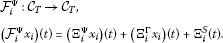(2.3)

Wherein,

$\begin{array}{c}\left({\mathrm{\Xi }}_{i}^{\mathrm{\Psi }}{x}_{i}\right)\left(t\right)={\int }_{0}^{T}{H}_{i}\left(t,s\right){\rho }_{i}\left(s\right){x}_{i}^{2}\left(s\right)\left[\frac{1}{{a}_{i}\left(s\right)+{\sum }_{j=1,j\ne i}^{n}{b}_{ij}\left(s\right){\int }_{-{\tau }_{ij}}^{0}{V}_{ij}\left(\theta \right){\xi }_{j}\left(s+\theta \right)\phantom{\rule{0.2em}{0ex}}d\theta }+{c}_{i}\left(s\right)\right]\phantom{\rule{0.2em}{0ex}}ds,\hfill \\ \left({\mathrm{\Xi }}_{i}^{\mathrm{\Gamma }}{x}_{i}\right)\left(t\right)={\int }_{0}^{T}{H}_{i}\left(t,s\right){x}_{i}\left(s\right)\left\{{d}_{i}\left(s\right)\left({\mathrm{\Gamma }}_{i}{x}_{i}\right)\left(s\right)+{e}_{i}\left(s\right){\int }_{-{\tau }_{i}}^{0}{W}_{i}\left(\theta \right)\left({\mathrm{\Gamma }}_{i}{x}_{i}\right)\left(s+\theta \right)\phantom{\rule{0.2em}{0ex}}d\theta \right\}\phantom{\rule{0.2em}{0ex}}ds,\hfill \end{array}$

and,

${\mathrm{\Xi }}_{i}^{S}\left(t\right)={\int }_{0}^{T}{H}_{i}\left(t,s\right){S}_{i}\left(s\right)\phantom{\rule{0.2em}{0ex}}ds.$

Kernel is given by

${H}_{i}\left(t,s\right)=\left\{\begin{array}{cc}\frac{1}{exp\left({\int }_{0}^{T}{\rho }_{i}\left(\theta \right)\phantom{\rule{0.2em}{0ex}}d\theta \right)-1}exp\left(-{\int }_{t}^{s}{\rho }_{i}\left(\theta \right)\phantom{\rule{0.2em}{0ex}}d\theta \right),\hfill & 0\le s\le t\le T,\hfill \\ \frac{{\int }_{0}^{T}{\rho }_{i}\left(\theta \right)\phantom{\rule{0.2em}{0ex}}d\theta \right)}{exp\left({\int }_{0}^{T}{\rho }_{i}\left(\theta \right)\phantom{\rule{0.2em}{0ex}}d\theta \right)-1}exp\left(-{\int }_{t}^{s}{\rho }_{i}\left(\theta \right)\phantom{\rule{0.2em}{0ex}}d\theta \right),\hfill & 0\le t\le s\le T.\hfill \end{array}$

Lemma 2.2 Let (${H}_{1}$) and (${H}_{2}$) hold and ${a}_{i}$, ${b}_{ij}$, ${c}_{i}$, ${d}_{i}$, ${\rho }_{i}$, ${\alpha }_{i}$, ${\beta }_{i}$, ${\gamma }_{i}$, $i,j=1,2,\dots ,N$ as well as x are all belong to ${\mathcal{C}}_{T}$ as well as ${\int }_{0}^{T}\rho \ne 0$. Then ${\mathcal{F}}_{i}^{\mathrm{\Psi }}x$ is T-periodic function and satisfies the following differential equation:

$\begin{array}{rcl}{\left({\mathcal{F}}_{i}^{\mathrm{\Psi }}x\right)}^{\prime }\left(t\right)& =& {\rho }_{i}\left(t\right)\left[\left({\mathcal{F}}_{i}^{\mathrm{\Psi }}x\right)\left(t\right)-\frac{{x}^{2}\left(t\right)}{{a}_{i}\left(t\right)+{\sum }_{j=1,j\ne i}^{n}{b}_{ij}\left(t\right){\int }_{-{\tau }_{ij}}^{0}{V}_{ij}\left(\theta \right){\xi }_{j}\left(t+\theta \right)\phantom{\rule{0.2em}{0ex}}d\theta }-{x}^{2}\left(t\right){c}_{i}\left(t\right)\right]\\ -x\left(t\right)\left\{{d}_{i}\left(t\right)\left({\mathrm{\Gamma }}_{i}x\right)\left(s\right)+{e}_{i}\left(t\right){\int }_{-{\tau }_{i}}^{0}{W}_{i}\left(\theta \right)\left({\mathrm{\Gamma }}_{i}x\right)\left(t+\theta \right)\phantom{\rule{0.2em}{0ex}}d\theta \right\}\\ -{S}_{i}\left(t\right).\end{array}$
(2.4)

Proof Appealing to presses of the proof of Lemma 2.1 for operators $\left({\mathrm{\Xi }}_{i}^{\mathrm{\Psi }}{x}_{i}\right)\left(t\right)$, $\left({\mathrm{\Xi }}_{i}^{\mathrm{\Gamma }}{x}_{i}\right)\left(t\right)$ and ${\mathrm{\Xi }}_{i}^{S}\left(t\right)$ one obtains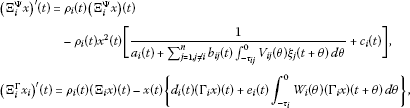and,

${\left({\mathrm{\Xi }}_{i}^{S}\right)}^{\prime }\left(t\right)={\rho }_{i}\left(t\right)\left({\mathrm{\Xi }}_{i}^{S}\right)\left(t\right)-{S}_{i}\left(s\right).$

The sum of terms above and taking the equality (2.3) into account leads to (2.4). □

Corollary 2.3 Let ${a}_{i}$, ${b}_{ij}$, ${c}_{i}$, ${d}_{i}$, ${\rho }_{i}$, ${\alpha }_{i}$, ${\beta }_{i}$, ${\gamma }_{i}$, $i,j=1,2,\dots ,n$ and x all belong to ${\mathcal{C}}_{T}$ as well as ${\int }_{0}^{T}\rho \ne 0$. Let that ${x}_{i}\in {\mathcal{C}}_{T}$ be a fixed point of the operator ${\mathcal{F}}_{i}^{\mathrm{\Psi }}$, i.e., ${\mathcal{F}}_{i}^{\mathrm{\Psi }}{x}_{i}={x}_{i}$, then ${x}_{i}$ is a solution of Eq. (2.2).

Theorem 2.4 Let

$\begin{array}{c}{\mathrm{\Lambda }}_{i}=\left\{\xi \in {\mathcal{C}}_{T}:\parallel \xi -{\mathrm{\Xi }}_{i}^{S}\parallel \le Q\parallel {\mathrm{\Xi }}_{i}^{S}\parallel \right\},\hfill \\ {Q}_{i}=\frac{{inf}_{t\in \left[0,T\right]}|{\mathrm{\Xi }}_{i}^{S}\left(t\right)|}{1+{sup}_{t\in \left[0,T\right]}|{\mathrm{\Xi }}_{i}^{S}\left(t\right)|},\phantom{\rule{2em}{0ex}}Q=min\left\{{Q}_{1},{Q}_{2},\dots ,{Q}_{N}\right\}.\hfill \end{array}$

Assume (${H}_{1}$) and (${H}_{2}$) hold, assume further that (${H}_{3}$) for $i=1,2,\dots ,N$

${J}_{i}\left(Q\right)+{A}_{i}{B}_{i}\le \left(1-\frac{1}{{\lambda }_{i}}\right)\left(1-\frac{1}{{\kappa }_{i}}\right)\frac{Q}{{\left(Q+1\right)}^{2}\parallel {\mathrm{\Xi }}_{i}^{S}\parallel }.$

Then the integral operator ${\mathcal{F}}_{i}^{\mathrm{\Psi }}$ maps ${\mathrm{\Lambda }}_{i}$ into ${\mathrm{\Lambda }}_{i}$ and has at least one fixed point.

Proof Let ${\xi }_{i}\in {\mathrm{\Lambda }}_{i}$, then

$|{\xi }_{i}\left(t\right)-{\mathrm{\Xi }}_{i}^{S}\left(t\right)|\le Q\parallel {\mathrm{\Xi }}_{i}^{S}\parallel ,$

thus

$|{\xi }_{i}\left(t\right)|\le \left(Q+1\right)\parallel {\mathrm{\Xi }}_{i}^{S}\parallel .$

On the other hand,

$\begin{array}{rcl}|{\xi }_{i}\left(t\right)|& \ge & |{\mathrm{\Xi }}_{i}^{S}\left(t\right)|-Q\parallel {\mathrm{\Xi }}_{i}^{S}\parallel \ge \underset{t\in \left[0,T\right]}{inf}|{\mathrm{\Xi }}_{i}^{S}\left(t\right)|-Q\underset{t\in \left[0,T\right]}{sup}|{\mathrm{\Xi }}_{i}^{S}\left(t\right)|\\ \ge & \underset{t\in \left[0,T\right]}{inf}|{\mathrm{\Xi }}_{i}^{S}\left(t\right)|-{Q}_{i}\underset{t\in \left[0,T\right]}{sup}|{\mathrm{\Xi }}_{i}^{S}\left(t\right)|\\ =& \underset{t\in \left[0,T\right]}{inf}|{\mathrm{\Xi }}_{i}^{S}\left(t\right)|-\frac{{inf}_{t\in \left[0,T\right]}|{\mathrm{\Xi }}_{i}^{S}\left(t\right)|{sup}_{t\in \left[0,T\right]}|{\mathrm{\Xi }}_{i}^{S}\left(t\right)|}{1+{sup}_{t\in \left[0,T\right]}|{\mathrm{\Xi }}_{i}^{S}\left(t\right)|}\\ =& {Q}_{i}\ge Q.\end{array}$

Consequently,

(2.5)

In addition, since ${\rho }_{i}$ and ${\alpha }_{i}$ ($i=1,2,\dots ,N$) are positive functions, we have

$\frac{1}{{\lambda }_{i}-1}\le {H}_{i}\left(t,s\right)\le \frac{{\lambda }_{i}}{{\lambda }_{i}-1},\phantom{\rule{1em}{0ex}}s,t\in \left[0,T\right],$

and

$\frac{1}{{\kappa }_{i}-1}\le {G}_{i}\left(t,s\right)\le \frac{{\kappa }_{i}}{{\kappa }_{i}-1},\phantom{\rule{1em}{0ex}}s,t\in \left[0,T\right].$

Therefore, for ${x}_{i}\in {\mathrm{\Lambda }}_{i}$, $\mathrm{\Psi }=\left({\xi }_{1},{\xi }_{2},\dots ,{\xi }_{N}\right)$ belong to ${⨂}_{i=1}^{N}{\mathrm{\Lambda }}_{i}$ and any $t\in \left[0,T\right]$, we obtain

$\begin{array}{rcl}|\left({\mathrm{\Xi }}_{i}^{\mathrm{\Psi }}{x}_{i}\right)\left(t\right)|& \le & \frac{{\lambda }_{i}}{{\lambda }_{i}-1}{\parallel {x}_{i}\parallel }^{2}\\ ×{\int }_{0}^{T}{\rho }_{i}\left(s\right)\left[\frac{1}{{a}_{i}\left(s\right)+{\sum }_{j=1,j\ne i}^{n}{b}_{ij}\left(s\right){\int }_{-{\tau }_{ij}}^{0}{V}_{ij}\left(\theta \right){\xi }_{j}\left(s+\theta \right)\phantom{\rule{0.2em}{0ex}}d\theta }+{c}_{i}\left(s\right)\right]\phantom{\rule{0.2em}{0ex}}ds\\ \le & \frac{{\lambda }_{i}}{{\lambda }_{i}-1}{\left(Q+1\right)}^{2}{\parallel {\mathrm{\Xi }}_{i}^{S}\parallel }^{2}{\int }_{0}^{T}{\rho }_{i}\left(s\right)\left[\frac{1}{{a}_{i}\left(s\right)+Q{\sum }_{j=1,j\ne i}^{n}{b}_{ij}\left(s\right)}+{c}_{i}\left(s\right)\right]\phantom{\rule{0.2em}{0ex}}ds\\ \le & \frac{{\lambda }_{i}}{{\lambda }_{i}-1}{\left(Q+1\right)}^{2}{\parallel {\mathrm{\Xi }}_{i}^{S}\parallel }^{2}{J}_{i}\left(Q\right).\end{array}$
(2.6)

Also, we have

$\begin{array}{rcl}|\left({\mathrm{\Gamma }}_{i}\xi \right)\left(t\right)|& \le & \frac{{\kappa }_{i}}{{\kappa }_{i}-1}\parallel {x}_{i}\parallel {\int }_{0}^{T}\left\{{\beta }_{i}\left(s\right)+{\gamma }_{i}\left(s\right){\int }_{-{\tau }_{i}}^{0}{U}_{i}\left(\theta \right)\phantom{\rule{0.2em}{0ex}}d\theta \right\}\phantom{\rule{0.2em}{0ex}}ds\\ \le & \frac{{\kappa }_{i}}{{\kappa }_{i}-1}\parallel {x}_{i}\parallel {A}_{i}.\end{array}$
(2.7)

Thus,

$\begin{array}{rcl}|\left({\mathrm{\Xi }}_{i}^{\mathrm{\Gamma }}{x}_{i}\right)\left(t\right)|& \le & \frac{{\lambda }_{i}}{{\lambda }_{i}-1}\parallel {x}_{i}\parallel {\int }_{0}^{T}\left\{{d}_{i}\left(s\right)\left({\mathrm{\Gamma }}_{i}{x}_{i}\right)\left(s\right)+{e}_{i}\left(s\right){\int }_{-{\tau }_{i}}^{0}{W}_{i}\left(\theta \right)\left({\mathrm{\Gamma }}_{i}{x}_{i}\right)\left(s+\theta \right)\phantom{\rule{0.2em}{0ex}}d\theta \right\}\phantom{\rule{0.2em}{0ex}}ds\\ \le & \frac{{\lambda }_{i}}{{\lambda }_{i}-1}\frac{{\kappa }_{i}}{{\kappa }_{i}-1}{\parallel {x}_{i}\parallel }^{2}{A}_{i}{\int }_{0}^{T}\left\{{d}_{i}\left(s\right)+{e}_{i}\left(s\right){\int }_{-{\tau }_{i}}^{0}{W}_{i}\left(\theta \right)\phantom{\rule{0.2em}{0ex}}d\theta \right\}\phantom{\rule{0.2em}{0ex}}ds\\ \le & \frac{{\lambda }_{i}{\kappa }_{i}}{\left({\lambda }_{i}-1\right)\left({\kappa }_{i}-1\right)}{\left(Q+1\right)}^{2}{\parallel {\mathrm{\Xi }}_{i}^{S}\parallel }^{2}{A}_{i}{B}_{i}.\end{array}$
(2.8)

In these regards, based on (2.6) and (2.8) one obtains

$\begin{array}{rcl}|\left({\mathcal{F}}_{i}^{\mathrm{\Psi }}{x}_{i}\right)\left(t\right)-{\mathrm{\Xi }}_{i}^{S}\left(t\right)|& =& |\left({\mathrm{\Xi }}_{i}^{\mathrm{\Psi }}{x}_{i}\right)\left(t\right)-\left({\mathrm{\Xi }}_{i}^{\mathrm{\Gamma }}{x}_{i}\right)\left(t\right)|\\ \le & |\left({\mathrm{\Xi }}_{i}^{\mathrm{\Psi }}{x}_{i}\right)\left(t\right)|+|\left({\mathrm{\Xi }}_{i}^{\mathrm{\Gamma }}{x}_{i}\right)\left(t\right)|\\ \le & \frac{{\lambda }_{i}}{{\lambda }_{i}-1}{\left(Q+1\right)}^{2}{\parallel {\mathrm{\Xi }}_{i}^{S}\parallel }^{2}\left({J}_{i}\left(Q\right)+\frac{{\kappa }_{i}}{{\kappa }_{i}-1}{A}_{i}{B}_{i}\right)\\ \le & \frac{{\lambda }_{i}{\kappa }_{i}}{\left({\lambda }_{i}-1\right)\left({\kappa }_{i}-1\right)}{\left(Q+1\right)}^{2}{\parallel {\mathrm{\Xi }}_{i}^{S}\parallel }^{2}\left({J}_{i}\left(Q\right)+{A}_{i}{B}_{i}\right)\\ \le & Q\parallel {\mathrm{\Xi }}_{i}^{S}\parallel .\end{array}$
(2.9)

This shows that ${\mathcal{F}}_{i}^{\mathrm{\Psi }}{x}_{i}$ is belong to ${\mathrm{\Lambda }}_{i}$ ($i=1,2,\dots ,N$) and, therefore, the integral operator ${\mathcal{F}}_{i}^{\mathrm{\Psi }}$ maps ${\mathrm{\Lambda }}_{i}$ into ${\mathrm{\Lambda }}_{i}$.

In addition, based on inequality (2.9), we have

$|\left({\mathcal{F}}_{i}^{\mathrm{\Psi }}{x}_{i}\right)\left(t\right)|\le \left(Q+1\right)\parallel {\mathrm{\Xi }}_{i}^{S}\parallel .$

Thus,

$\begin{array}{rcl}|{\left({\mathcal{F}}_{i}^{\mathrm{\Psi }}x\right)}^{\prime }\left(t\right)|& \le & \parallel {\rho }_{i}\parallel \left(Q+1\right)\parallel {\mathrm{\Xi }}_{i}^{S}\parallel +{\left(Q+1\right)}^{2}{\parallel {\mathrm{\Xi }}_{i}^{S}\parallel }^{2}\\ ×\left\{{I}_{i}\left(Q\right)+\frac{{\kappa }_{i}}{{\kappa }_{i}-1}{A}_{i}\left\{\parallel {d}_{i}\parallel +\parallel {e}_{i}\parallel \right\}\right\}+\parallel {S}_{i}\parallel ,\end{array}$
(2.10)

which shows that $|{\left({\mathcal{F}}_{i}^{\mathrm{\Psi }}x\right)}^{\prime }\left(t\right)|$ is bounded by

$\begin{array}{rcl}{M}_{i}& =& \parallel {\rho }_{i}\parallel \left(Q+1\right)\parallel {\mathrm{\Xi }}_{i}^{S}\parallel \\ +{\left(Q+1\right)}^{2}{\parallel {\mathrm{\Xi }}_{i}^{S}\parallel }^{2}\left\{{I}_{i}\left(Q\right)+\frac{{\kappa }_{i}}{{\kappa }_{i}-1}{A}_{i}\left\{\parallel {d}_{i}\parallel +\parallel {e}_{i}\parallel \right\}\right\}+\parallel {S}_{i}\parallel .\end{array}$

Let $M=max\left\{{M}_{1},{M}_{2},\dots ,{M}_{N}\right\}$. For any $t,s\in \left[0,T\right]$, one obtains

$|\left({\mathcal{F}}_{i}^{\mathrm{\Psi }}x\right)\left(t\right)-\left({\mathcal{F}}_{i}^{\mathrm{\Psi }}x\right)\left(s\right)|\le M|t-s|.$

This implies that $\left({\mathcal{F}}_{i}^{\mathrm{\Psi }}x\right)\left(t\right)$ is Lipschitzan with Lipschit constance M. In this way, for given $\epsilon >0$, if we consider $\delta \left(\epsilon \right)=\frac{\epsilon }{M}$, then

Consequently, for any $1\le i\le N$ the family ${\left\{{\mathcal{F}}_{i}^{\mathrm{\Psi }}x\right\}}_{x\in {\mathrm{\Lambda }}_{i}}$ is equicontinuous on $\left[0,T\right]$.

Suppose $\left\{{x}_{i,m}\right\}$ is a sequence on ${\mathrm{\Lambda }}_{i}$, $1\le i\le N$. Thus, $\left\{\left({\mathcal{F}}_{i}^{\mathrm{\Psi }}{x}_{i,m}\right)\left(t\right)\right\}$ as a sequence of functions on $\left[0,T\right]$ is equicontinuous. Appealing to the Arzela-Ascoli theorem, there exist a subsequent denoted by $\left\{\left({\mathcal{F}}_{i}^{\mathrm{\Psi }}{x}_{i,{m}_{k}}\right)\left(t\right)\right\}$, which is uniformly convergence on $\left[0,T\right]$. This means that $\left\{{\mathcal{F}}_{i}^{\mathrm{\Psi }}{x}_{i,{m}_{k}}\right\}$ is convergent on ${\mathrm{\Lambda }}_{i}$ and consequently, ${\mathcal{F}}_{i}^{\mathrm{\Psi }}:{\mathrm{\Lambda }}_{i}\to {\mathrm{\Lambda }}_{i}$ is compact. Appealing to Schauder’s fixed-point theorem, ${\mathcal{F}}_{i}^{\mathrm{\Psi }}$ has at least a fixed point on ${\mathrm{\Lambda }}_{i}$. □

We emphasize that according to Corollary 2.3, for any $1\le i\le N$, and $\mathrm{\Psi }=\left({\xi }_{1},{\xi }_{2},\dots ,{x}_{i},\dots ,{\xi }_{N}\right)$ belong to ${⨂}_{i=1}^{N}{\mathrm{\Lambda }}_{i}$ fixed point of ${\mathcal{F}}_{i}^{\mathrm{\Psi }}$ is the positive T-periodic solutions of Eq. (2.2) or equivalently, T-periodic solution of the nonlinear population system (1.3) and (1.4).

Let $\mathrm{\Psi }=\left({\xi }_{1},{\xi }_{2},\dots ,{\xi }_{N}\right)\in {⨂}_{i=1}^{N}{\mathrm{\Lambda }}_{i}$ and (${H}_{1}$), (${H}_{2}$), and (${H}_{3}$) hold. Based on Theorem 2.4 for $i=1,2,\dots ,N$, operator ${\mathcal{F}}_{i}^{\mathrm{\Psi }}$ has at least a fixed point in ${\mathrm{\Lambda }}_{i}$. Let $Fix\left({\mathcal{F}}_{i}^{\mathrm{\Psi }}\right)\subseteq {\mathrm{\Lambda }}_{i}$ denote the set of fixed points of ${\mathcal{F}}_{i}^{\mathrm{\Psi }}$. Applying the Axiom of Choice, we chose a representative point, say ${x}_{i\mathrm{\Psi }}$, in $Fix\left({\mathcal{F}}_{i}^{\mathrm{\Psi }}\right)$ i.e.,

$\left({\mathcal{F}}_{i}^{\mathrm{\Psi }}{x}_{i\mathrm{\Psi }}\right)\left(t\right)={x}_{i\mathrm{\Psi }}\left(t\right),\phantom{\rule{1em}{0ex}}t\in \left[0,T\right],i=1,2,\dots ,N.$

We introduce the following operator on ${⨂}_{i=1}^{N}{\mathrm{\Lambda }}_{i}$: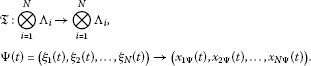(2.11)

Theorem 2.5 Let (${H}_{1}$), (${H}_{2}$), and (${H}_{3}$) hold. Then operator $\mathfrak{T}:{⨂}_{i=1}^{N}{\mathrm{\Lambda }}_{i}\to {⨂}_{i=1}^{N}{\mathrm{\Lambda }}_{i}$ defined by (2.11) has at least a fixed point on ${⨂}_{i=1}^{N}{\mathrm{\Lambda }}_{i}$.

Proof It is obvious that $\mathfrak{T}$ is bounded on B. Also, according to inequality (2.10), we have

$|{x}_{i\mathrm{\Psi }}^{\prime }\left(t\right)|\le M,\phantom{\rule{1em}{0ex}}t\in \left[0,T\right],i=1,2,\dots ,N.$

Thus,

$|{x}_{i\mathrm{\Psi }}\left(t\right)-{x}_{i\mathrm{\Psi }}\left(s\right)|\le M|t-s|,\phantom{\rule{1em}{0ex}}t,s\in \left[0,T\right],i=1,2,\dots ,N.$

For given $\epsilon >0$, if we consider $\delta \left(\epsilon \right)=\frac{\epsilon }{MN}$, then

which shows that $\mathfrak{T}\left({⨂}_{i=1}^{N}{\mathrm{\Lambda }}_{i}\right)$ is equicontinuous on [0,T]. In this regard, any sequence, say $\left\{{X}_{k}\right\}$ in ${⨂}_{i=1}^{N}{\mathrm{\Lambda }}_{i}$ satisfies all the conditions of the Arzela-Ascoli theorem on [0,T]. Hence, $\left\{{X}_{k}\right\}$ has a subsequence $\left\{{X}_{{k}_{m}}\right\}$ such that is uniformly convergent on [0,T]. This shows that $\mathfrak{T}\left({⨂}_{i=1}^{N}{\mathrm{\Lambda }}_{i}\right)$ is relatively compact in B.

In the sequel, we show that $\mathfrak{T}$ is continuous. We define the following map on ${⨂}_{i=1}^{N}{\mathrm{\Lambda }}_{i}×\left[0,T\right]$,

$\begin{array}{rcl}{Z}_{i}\left(\mathrm{\Psi },t\right)& =& {\rho }_{i}\left(t\right)\left[1-\frac{{\xi }_{i}\left(t\right)}{{a}_{i}\left(t\right)+{\sum }_{j=1,j\ne i}^{n}{b}_{ij}\left(t\right){\int }_{-{\tau }_{ij}}^{0}{V}_{ij}\left(\theta \right){\xi }_{j}\left(t+\theta \right)\phantom{\rule{0.2em}{0ex}}d\theta }-{c}_{i}\left(t\right){\xi }_{i}\left(t\right)\right]\\ -{d}_{i}\left(t\right)\left({\mathrm{\Gamma }}_{i}{\xi }_{i}\right)\left(t\right)-{e}_{i}\left(t\right){\int }_{-{\tau }_{i}}^{0}{W}_{i}\left(\theta \right)\left({\mathrm{\Gamma }}_{i}{\xi }_{i}\right)\left(t+\theta \right)\phantom{\rule{0.2em}{0ex}}d\theta .\end{array}$
(2.12)

Wherein, $\mathrm{\Psi }=\left({\xi }_{1},{\xi }_{2},\dots ,{\xi }_{N}\right)$. For each $1\le i,j\le N$, the partial derivative $\partial {Z}_{i}\left(\mathrm{\Psi },t\right)/\partial {\xi }_{j}$ exist and straightforward calculation shows

$\begin{array}{rcl}|\frac{\partial {Z}_{i}\left(\mathrm{\Psi },t\right)}{\partial {\xi }_{i}}|& \le & |{\rho }_{i}\left(t\right)|\left\{|\frac{1}{{a}_{i}\left(t\right)+{\sum }_{j=1,j\ne i}^{n}{b}_{ij}\left(t\right){\int }_{-{\tau }_{ij}}^{0}{V}_{ij}\left(\theta \right){\xi }_{j}\left(t+\theta \right)\phantom{\rule{0.2em}{0ex}}d\theta }|+|{c}_{i}\left(t\right)|\right\}\\ +|{d}_{i}\left(t\right){\int }_{0}^{T}{G}_{i}\left(t,s\right)\left\{{\beta }_{i}\left(s\right)+{\gamma }_{i}\left(s\right)\right\}\phantom{\rule{0.2em}{0ex}}ds|\\ +|{e}_{i}\left(t\right){\int }_{-{\tau }_{i}}^{0}{W}_{i}\left(\theta \right)\left\{{\int }_{0}^{T}{G}_{i}\left(t,s\right)\left\{{\beta }_{i}\left(s\right)+{\gamma }_{i}\left(s\right)\right\}\phantom{\rule{0.2em}{0ex}}ds\right\}\phantom{\rule{0.2em}{0ex}}d\theta |\\ \le & \parallel {\rho }_{i}\parallel {I}_{i}\left(Q\right)+\frac{{\kappa }_{i}}{{\kappa }_{i}-1}{A}_{i}\left\{\parallel {d}_{i}\parallel +\parallel {e}_{i}\parallel \right\}.\end{array}$
(2.13)

Also, in the case $k\ne i$,

$\begin{array}{rcl}|\frac{\partial {Z}_{i}\left(\mathrm{\Psi },t\right)}{\partial {\xi }_{k}}|& \le & \frac{{\rho }_{i}\left(t\right){\xi }_{k}\left(t\right){b}_{ik}\left(t\right)}{{\left({a}_{i}\left(t\right)+{\sum }_{j=1,j\ne i}^{n}{b}_{ij}\left(t\right){\int }_{-{\tau }_{ij}}^{0}{V}_{ij}\left(\theta \right){\xi }_{j}\left(t+\theta \right)\phantom{\rule{0.2em}{0ex}}d\theta \right)}^{2}}\\ \le & \left(Q+1\right)\parallel {\rho }_{i}{b}_{ik}{\mathrm{\Xi }}_{i}^{S}\parallel {\left({I}_{i}\left(Q\right)-\parallel {c}_{i}\parallel \right)}^{2}.\end{array}$
(2.14)

We set $\sigma =max\left\{{g}_{i},{h}_{ij}:i,j=1,2,\dots ,N\right\}$, wherein,

${g}_{i}=\parallel {\rho }_{i}\parallel {I}_{i}\left(Q\right)+\frac{{\kappa }_{i}}{{\kappa }_{i}-1}{A}_{i}\left\{\parallel {d}_{i}\parallel +\parallel {e}_{i}\parallel \right\},$

and,

${h}_{ij}=\left(Q+1\right)\parallel {\rho }_{i}{b}_{ik}{\mathrm{\Xi }}_{i}^{S}\parallel {\left({I}_{i}\left(Q\right)-\parallel {c}_{i}\parallel \right)}^{2}.$

Therefore,

(2.15)

Based on property (2.15) and by induction, one can obtain

$|{Z}_{i}\left({\xi }_{1},{\xi }_{2},\dots ,{\xi }_{N},t\right)-{Z}_{i}\left({\varphi }_{1},{\varphi }_{2},\dots ,{\varphi }_{N},t\right)|\le P\left(\sigma \right)\sum _{i=1}^{N}|{\xi }_{i}\left(t\right)-{\varphi }_{i}\left(t\right)|,$
(2.16)

where $P\left(\sigma \right)$ is a specific polynomial in terms of σ.

Let$\left\{{\mathrm{\Psi }}_{k}\right\}$ be a sequence belong to ${⨂}_{i=1}^{N}{\mathrm{\Lambda }}_{i}$ and ${\mathrm{\Psi }}_{k}=\left({\xi }_{1k},{\xi }_{2k},\dots ,{\xi }_{Nk}\right)\to \mathrm{\Phi }=\left({\varphi }_{1},{\varphi }_{2},\dots ,{\varphi }_{N}\right)$ as $k↦\mathrm{\infty }$. Let $\mathfrak{T}{\mathrm{\Psi }}_{k}={X}_{{\mathrm{\Psi }}_{k}}=\left({x}_{1{\mathrm{\Psi }}_{k}},{x}_{2{\mathrm{\Psi }}_{k}},\dots ,{x}_{N{\mathrm{\Psi }}_{k}}\right)\in \mathfrak{T}\left({⨂}_{i=1}^{N}{\mathrm{\Lambda }}_{i}\right)$. According to relative compactness of ${⨂}_{i=1}^{N}{\mathrm{\Lambda }}_{i}$ in B, there exist a subsequence $\left\{{X}_{{\mathrm{\Psi }}_{q}}\right\}$ of $\left\{{X}_{{\mathrm{\Psi }}_{k}}\right\}$ and $Y=\left({y}_{1},{y}_{2},\dots ,{y}_{N}\right)\in B$ such that ${X}_{{\mathrm{\Psi }}_{q}}↦Y$, uniformly, in B as $q↦\mathrm{\infty }$. It is obvious that for any q, we have

$\begin{array}{rcl}\frac{d{x}_{i{\mathrm{\Psi }}_{q}}}{dt}& =& {x}_{i{\mathrm{\Psi }}_{q}}\left(t\right){\rho }_{i}\left(t\right)\left[1-\frac{{x}_{i{\mathrm{\Psi }}_{q}}\left(t\right)}{{a}_{i}\left(t\right)+{\sum }_{j=1,j\ne i}^{n}{b}_{ij}\left(t\right){\int }_{-{\tau }_{ij}}^{0}{V}_{ij}\left(\theta \right){\xi }_{jq}\left(t+\theta \right)\phantom{\rule{0.2em}{0ex}}d\theta }-{c}_{i}\left(t\right){x}_{i{\mathrm{\Psi }}_{q}}\left(t\right)\right]\\ -{d}_{i}\left(t\right)\left({\mathrm{\Gamma }}_{i}{x}_{i{\mathrm{\Psi }}_{q}}\right)\left(t\right){x}_{i{\mathrm{\Psi }}_{q}}\left(t\right)-{e}_{i}\left(t\right){x}_{i{\mathrm{\Psi }}_{q}}\left(t\right){\int }_{-{\tau }_{i}}^{0}{W}_{i}\left(\theta \right)\left({\mathrm{\Gamma }}_{i}{x}_{i{\mathrm{\Psi }}_{q}}\right)\left(t+\theta \right)\phantom{\rule{0.2em}{0ex}}d\theta -{S}_{i}\left(t\right)\\ =& {x}_{i{\mathrm{\Psi }}_{q}}\left(t\right){Z}_{i}\left({\xi }_{1q}\left(t\right),\dots ,{\xi }_{i-1q}\left(t\right),{x}_{i{\mathrm{\Psi }}_{q}}\left(t\right),{\xi }_{i+1q}\left(t\right),\dots ,{\xi }_{Nq}\left(t\right),t\right).\end{array}$
(2.17)

Or equivalently,

$\left({\mathcal{F}}_{i}^{{\mathrm{\Psi }}_{q}}{x}_{i{\mathrm{\Psi }}_{q}}\right)\left(t\right)={x}_{i{\mathrm{\Psi }}_{q}}\left(t\right),\phantom{\rule{1em}{0ex}}t\in \left[0,T\right],i=1,2,\dots ,N.$

Based on property (2.16), we have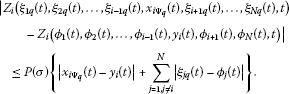(2.18)

Therefore,

${x}_{i{\mathrm{\Psi }}_{q}}\left(t\right){Z}_{i}\left({\xi }_{1q}\left(t\right),{\xi }_{2q}\left(t\right),\dots ,{\xi }_{i-1q}\left(t\right),{x}_{i{\mathrm{\Psi }}_{q}}\left(t\right),{\xi }_{i+1q}\left(t\right),\dots ,{\xi }_{Nq}\left(t\right),t\right)$

converges to

${y}_{i}\left(t\right){Z}_{i}\left({\varphi }_{1}\left(t\right),{\varphi }_{2}\left(t\right),\dots ,{\varphi }_{i-1}\left(t\right),{y}_{i}\left(t\right),{\varphi }_{i+1}\left(t\right),{\varphi }_{N}\left(t\right),t\right)$

uniformly, as $q↦\mathrm{\infty }$ for all $t\in \left[0,T\right]$ and $i=1,2,\dots ,N$.

Thus, derivative $d{y}_{i}/dt$ exist on $\left[0,T\right]$ and

Or equivalently,

$d{y}_{i}/dt={y}_{i}\left(t\right){Z}_{i}\left({\varphi }_{1}\left(t\right),{\varphi }_{2}\left(t\right),\dots ,{\varphi }_{i-1}\left(t\right),{y}_{i}\left(t\right),{\varphi }_{i+1}\left(t\right),{\varphi }_{N}\left(t\right),t\right)$

for all $t\in \left[0,T\right]$ and $i=1,2,\dots ,N$.

Consequently, based on definition of map $\mathfrak{T}$ we have $\mathfrak{T}\mathrm{\Phi }=Y$. Also, ${\mathrm{\Psi }}_{q}↦\mathrm{\Phi }$. Thus, we obtain

$\underset{q↦\mathrm{\infty }}{lim}\mathfrak{T}{\mathrm{\Psi }}_{q}=Y=\mathfrak{T}\mathrm{\Phi }=\mathfrak{T}\underset{q↦\mathrm{\infty }}{lim}{\mathrm{\Phi }}_{q}.$

Hence, ${lim}_{k↦\mathrm{\infty }}\mathfrak{T}{\mathrm{\Psi }}_{k}=Y$, which it shows that $\mathfrak{T}$ is continuous on ${⨂}_{i=1}^{N}{\mathrm{\Lambda }}_{i}$.

Therefore, applying Schauder’s fixed-point theorem, map $\mathfrak{T}$ has a fixed point $\mathrm{\Phi }=\left({\varphi }_{1}\left(t\right),\dots ,{\varphi }_{N}\left(t\right)\right)\in {⨂}_{i=1}^{N}{\mathrm{\Lambda }}_{i}$, i.e.,

$\mathfrak{T}\mathrm{\Phi }=\mathrm{\Phi }.$

Or equivalently,

$\left({\mathcal{F}}_{i}^{\mathrm{\Phi }}{\varphi }_{i}\right)\left(t\right)={\varphi }_{i}\left(t\right),\phantom{\rule{1em}{0ex}}t\in \left[0,T\right],i=1,2,\dots ,N.$

Finally, this indicate that $\left({\varphi }_{1}\left(t\right),\dots ,{\varphi }_{N}\left(t\right)\right)$ is a positive periodic solution of system of Eq. (2.2) or equivalently, T-periodic solution of the multi-species cooperation system (1.3) and (1.4). This completes the proof of the theorem. □

### 2.1 Permanence of system

Let $\tau =\left\{{\tau }_{ij},{\tau }_{i},{\eta }_{i},i,j=1,2,\dots ,N\right\}$. We consider the system (1.4), (1.5) together with the following initial conditions: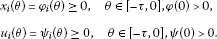(2.19)

Lemma 2.6 (see )

If $a>0$, $b>0$ and $\stackrel{˙}{u}\ge b-au$, when $t\ge {t}_{0}$ and $u\left({t}_{0}\right)>0$, we have

$u\left(t\right)\ge \frac{b}{a}{\left[1+\left(\frac{au\left({t}_{0}\right)}{b}-1\right)exp\left(-a\left(t-{t}_{0}\right)\right)\right]}^{-1}.$

Theorem 2.7 Let (${H}_{1}$), (${H}_{2}$), (${H}_{3}$) hold and $\left({x}_{1},{x}_{2},\dots ,{x}_{N}\right)\in {⨂}_{i=1}^{N}{\mathrm{\Lambda }}_{i}$ be any solution differential equation (2.2) and $\left({u}_{1},{u}_{2},\dots ,{u}_{N}\right)$ with

${u}_{i}\left(t\right)={\int }_{0}^{T}{G}_{i}\left(t,s\right)\left\{{\beta }_{i}\left(s\right)\xi \left(s\right)+{\gamma }_{i}\left(s\right){\int }_{-{\tau }_{i}}^{0}{U}_{i}\left(\theta \right)\xi \left(s+\theta \right)\phantom{\rule{0.2em}{0ex}}d\theta \right\}\phantom{\rule{0.2em}{0ex}}ds,$
(2.20)

be the solution of system (1.5) with initial condition (2.19). Then solution $\left({x}_{1},{x}_{2},\dots ,{x}_{N},{u}_{1},{u}_{2},\dots ,{u}_{N}\right)$ of system (1.4), (1.5) is permanent, i.e., there exist $\stackrel{˜}{\tau }>0$ and ${m}_{i}>0$, ${M}_{i}$, ${\stackrel{˜}{m}}_{i}>0$, and ${\stackrel{˜}{M}}_{i}$ ($i=1,2,\dots ,N$) such that

${m}_{i}\le {x}_{i}\left(t\right)\le {M}_{i},\phantom{\rule{2em}{0ex}}{\stackrel{˜}{m}}_{i}\le {u}_{i}\left(t\right)\le {\stackrel{˜}{M}}_{i}.$

Proof Since ${S}_{i}\ge 0$ for any $0\le i\le N$, appealing to the proof of the Lemma 2.3 given in  we can immediately demonstrate that there exist a $\stackrel{˜}{\tau }>0$ such that

(2.21)

where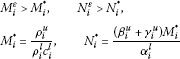(2.22)

while ${f}^{u}={sup}_{t}f\left(t\right)$ and ${f}^{l}={inf}_{t}f\left(t\right)$.

On the other hand, from Lemma 2.1 and inequality (2.5), we have

$\begin{array}{rcl}\frac{d{u}_{i}}{dt}& =& -{\alpha }_{i}\left(t\right){u}_{i}\left(t\right)+{\beta }_{i}\left(t\right){x}_{i}\left(t\right)+{\gamma }_{i}\left(t\right){\int }_{-{\eta }_{i}}^{0}{U}_{i}\left(\theta \right){x}_{i}\left(t+\theta \right)\phantom{\rule{0.2em}{0ex}}d\theta \\ \le & -{\alpha }_{i}^{u}+\left({\beta }_{i}^{l}+{\gamma }_{i}^{l}\right)Q,\end{array}$

then by Lemma 2.6, for arbitrary $ϵ>0$, there exist ${\tau }^{\prime }>\stackrel{˜}{\tau }$ such that

Since ϵ is arbitrary small, one may assume that

$ϵ\le \frac{\left({\beta }_{i}^{l}+{\gamma }_{i}^{l}\right)Q}{{\alpha }_{i}^{u}}.$

Thus,

□

Remark 2.8 For the system without the external source, the sets ${\mathrm{\Lambda }}_{i}$ must be replaced by

${B}_{R}=\left\{\xi \in {\mathcal{C}}_{T}:\parallel \xi \parallel \le R\right\}.$

Similar calculation shows that condition (${H}_{3}$) is reduced to the following one:

$\left(\frac{{\lambda }_{i}}{{\lambda }_{i}-1}\right)\left[{J}_{i}\left(0\right)+\left(\frac{{\kappa }_{i}}{{\kappa }_{i}-1}\right){A}_{i}{B}_{i}\right]\le \frac{1}{R}.$

## 3 Illustrative example

Consider the following 2-species cooperation system with delay, feedback control, and external source

$\begin{array}{rcl}\frac{d{N}_{1}}{dt}& =& {\rho }_{1}\left(t\right){N}_{1}\left(t\right)\left[1-\frac{{N}_{1}\left(t\right)}{{a}_{1}\left(t\right)+{b}_{12}\left(t\right){\int }_{-{\tau }_{12}}^{0}{V}_{12}\left(\theta \right){N}_{2}\left(t+\theta \right)\phantom{\rule{0.2em}{0ex}}d\theta }-{c}_{1}\left(t\right){N}_{1}\left(t\right)\right]\\ -{d}_{1}\left(t\right){u}_{1}\left(t\right){N}_{1}\left(t\right)-{e}_{1}\left(t\right){N}_{1}\left(t\right){\int }_{-{\tau }_{1}}^{0}{W}_{1}\left(\theta \right){u}_{1}\left(t+\theta \right)\phantom{\rule{0.2em}{0ex}}d\theta -{S}_{1}\left(t\right),\end{array}$
(3.1)
$\frac{d{u}_{1}}{dt}=-{\alpha }_{1}\left(t\right){u}_{1}\left(t\right)+{\beta }_{1}\left(t\right){N}_{1}\left(t\right)+{\gamma }_{1}\left(t\right){\int }_{-{\tau }_{1}}^{0}{U}_{1}\left(\theta \right){N}_{1}\left(t+\theta \right)\phantom{\rule{0.2em}{0ex}}d\theta ,$
(3.2)
$\begin{array}{rcl}\frac{d{N}_{2}}{dt}& =& {\rho }_{2}\left(t\right){N}_{2}\left(t\right)\left[1-\frac{{N}_{2}\left(t\right)}{{a}_{2}\left(t\right)+{b}_{21}\left(t\right){\int }_{-{\tau }_{21}}^{0}{V}_{21}\left(\theta \right){N}_{1}\left(t+\theta \right)\phantom{\rule{0.2em}{0ex}}d\theta }-{c}_{2}\left(t\right){N}_{2}\left(t\right)\right]\\ -{d}_{2}\left(t\right){u}_{2}\left(t\right){N}_{2}\left(t\right)-{e}_{2}\left(t\right){N}_{2}\left(t\right){\int }_{-{\tau }_{2}}^{0}{W}_{2}\left(\theta \right){u}_{2}\left(t+\theta \right)\phantom{\rule{0.2em}{0ex}}d\theta -{S}_{2}\left(t\right),\end{array}$
(3.3)
$\frac{d{u}_{2}}{dt}=-{\alpha }_{2}\left(t\right){u}_{2}\left(t\right)+{\beta }_{2}\left(t\right){N}_{2}\left(t\right)+{\gamma }_{2}\left(t\right){\int }_{-{\tau }_{2}}^{0}{U}_{2}\left(\theta \right){N}_{2}\left(t+\theta \right)\phantom{\rule{0.2em}{0ex}}d\theta .$
(3.4)

Wherein,

$\begin{array}{c}{\rho }_{1}\left(t\right)=\frac{5-sin\left(2\pi t\right)-cos\left(4\pi t\right)}{5-sin\left(2\pi t\right)+cos\left(2\pi t\right)},\phantom{\rule{2em}{0ex}}{\rho }_{2}\left(t\right)=\frac{7-sin\left(2\pi t\right)-cos\left(4\pi t\right)}{6-3sin\left(2\pi t\right)+cos\left(2\pi t\right)},\hfill \\ {S}_{1}\left(t\right)=\frac{2-sin\left(2\pi t\right)}{2+sin\left(2\pi t\right)},\phantom{\rule{2em}{0ex}}{S}_{2}\left(t\right)=\frac{2-sin\left(2\pi t\right)}{2+cos\left(2\pi t\right)},\hfill \\ {H}_{1}\left(t,s\right)=\left\{\begin{array}{cc}0.5270exp\left({\int }_{s}^{t}{\rho }_{1}\left(\theta \right)\phantom{\rule{0.2em}{0ex}}d\theta \right),\hfill & 0\le s\le t\le T,\hfill \\ 1.5270exp\left({\int }_{s}^{t}{\rho }_{1}\left(\theta \right)\phantom{\rule{0.2em}{0ex}}d\theta \right),\hfill & 0\le t\le s\le T,\hfill \end{array}\hfill \\ {H}_{2}\left(t,s\right)=\left\{\begin{array}{cc}0.3110exp\left({\int }_{s}^{t}{\rho }_{2}\left(\theta \right)\phantom{\rule{0.2em}{0ex}}d\theta \right),\hfill & 0\le s\le t\le T,\hfill \\ 1.3110exp\left({\int }_{s}^{t}{\rho }_{2}\left(\theta \right)\phantom{\rule{0.2em}{0ex}}d\theta \right),\hfill & 0\le t\le s\le T,\hfill \end{array}\hfill \\ {\alpha }_{1}\left(t\right)=\frac{15+sin\left(2\pi t\right)+cos\left(2\pi t\right)}{10-sin\left(2\pi t\right)-cos\left(2\pi t\right)},\phantom{\rule{1em}{0ex}}{\alpha }_{2}\left(t\right)=\frac{12+sin\left(2\pi t\right)-cos\left(2\pi t\right)}{5-sin\left(2\pi t\right)-cos\left(2\pi t\right)},\hfill \\ {G}_{1}\left(t,s\right)=\left\{\begin{array}{cc}1.2813exp\left({\int }_{t}^{s}{\alpha }_{1}\left(\theta \right)\phantom{\rule{0.2em}{0ex}}d\theta \right),\hfill & 0\le s\le t\le T,\hfill \\ 0.2813exp\left({\int }_{t}^{s}{\alpha }_{1}\left(\theta \right)\phantom{\rule{0.2em}{0ex}}d\theta \right),\hfill & 0\le t\le s\le T,\hfill \end{array}\hfill \\ {G}_{2}\left(t,s\right)=\left\{\begin{array}{cc}1.0893exp\left({\int }_{t}^{s}{\alpha }_{2}\left(\theta \right)\phantom{\rule{0.2em}{0ex}}d\theta \right),\hfill & 0\le s\le t\le T,\hfill \\ 0.0893exp\left({\int }_{t}^{s}{\alpha }_{2}\left(\theta \right)\phantom{\rule{0.2em}{0ex}}d\theta \right),\hfill & 0\le t\le s\le T,\hfill \end{array}\hfill \\ \underset{t\in \left[0,T\right]}{sup}|{\mathrm{\Xi }}_{1}^{S}\left(t\right)|=1.9994,\phantom{\rule{2em}{0ex}}\underset{t\in \left[0,T\right]}{sup}|{\mathrm{\Xi }}_{2}^{S}\left(t\right)|=1.5138,\hfill \\ \underset{t\in \left[0,T\right]}{inf}|{\mathrm{\Xi }}_{1}^{S}\left(t\right)|=0.69,\phantom{\rule{2em}{0ex}}\underset{t\in \left[0,T\right]}{inf}|{\mathrm{\Xi }}_{2}^{S}\left(t\right)|=0.3591,\hfill \\ {\lambda }_{1}=2.8975,\phantom{\rule{1em}{0ex}}{\lambda }_{2}=4.2146,\phantom{\rule{1em}{0ex}}{\kappa }_{1}=4.5540,\phantom{\rule{1em}{0ex}}{\kappa }_{2}=12.2089,\hfill \\ {Q}_{1}=0.23,\phantom{\rule{2em}{0ex}}{Q}_{2}=0.1428,\phantom{\rule{2em}{0ex}}Q=0.23,\hfill \\ {a}_{1}\left(t\right)=100+sin\left(2\pi t\right),\phantom{\rule{2em}{0ex}}{a}_{2}\left(t\right)=220-cos\left(2\pi t\right),\hfill \\ {c}_{1}\left(t\right)=\frac{2+sin\left(2\pi t\right)}{200+cos\left(2\pi t\right)},\phantom{\rule{2em}{0ex}}{c}_{2}\left(t\right)=\frac{2-cos\left(2\pi t\right)}{200-sin\left(2\pi t\right)},\hfill \\ {b}_{12}\left(t\right)=30+sin\left(2\pi t\right),\phantom{\rule{2em}{0ex}}{b}_{21}\left(t\right)=100-cos\left(2\pi t\right),\hfill \\ {J}_{1}\left(Q\right)=0.02167,\phantom{\rule{2em}{0ex}}{J}_{2}\left(Q\right)=0.02171,\hfill \\ {\beta }_{1}\left(t\right)=\frac{3-sin\left(2\pi t\right)-cos\left(4\pi t\right)}{10+sin\left(4\pi t\right)+cos\left(4\pi t\right)},\phantom{\rule{2em}{0ex}}{\beta }_{2}\left(t\right)=\frac{3-cos\left(4\pi t\right)}{32+2sin\left(2\pi t\right)+cos\left(2\pi t\right)},\hfill \\ {\gamma }_{1}\left(t\right)=\frac{7-sin\left(2\pi t\right)+cos\left(4\pi t\right)}{30+2sin\left(4\pi t\right)+cos\left(4\pi t\right)},\phantom{\rule{2em}{0ex}}{\gamma }_{2}\left(t\right)=\frac{2+sin\left(4\pi t\right)}{29-2sin\left(2\pi t\right)+3cos\left(2\pi t\right)},\hfill \\ {d}_{1}\left(t\right)=\frac{2-sin\left(4\pi t\right)}{21+sin\left(4\pi t\right)+cos\left(8\pi t\right)},\phantom{\rule{2em}{0ex}}{d}_{2}\left(t\right)=\frac{3+sin\left(4\pi t\right)}{40+sin\left(2\pi t\right)-2cos\left(2\pi t\right)},\hfill \\ {e}_{1}\left(t\right)=\frac{4+sin\left(2\pi t\right)}{20-sin\left(2\pi t\right)+cos\left(4\pi t\right)},\phantom{\rule{2em}{0ex}}{e}_{2}\left(t\right)=\frac{2-cos\left(4\pi t\right)}{72+sin\left(2\pi t\right)+4cos\left(2\pi t\right)},\hfill \\ {A}_{1}=0.5411,\phantom{\rule{2em}{0ex}}{B}_{1}=0.2970,\phantom{\rule{2em}{0ex}}{A}_{2}=0.1633,\phantom{\rule{2em}{0ex}}{B}_{1}=0.1029.\hfill \end{array}$

With due attention to the data above, we have

${J}_{1}\left(Q\right)+{A}_{1}{B}_{1}=0.1823\le \left(1-\frac{1}{{\lambda }_{1}}\right)\left(1-\frac{1}{{\kappa }_{1}}\right)\frac{Q}{{\left(Q+1\right)}^{2}\parallel {\mathrm{\Xi }}_{1}^{S}\parallel }=0.5123,$

and

${J}_{2}\left(Q\right)+{A}_{2}{B}_{2}=0.0385\le \left(1-\frac{1}{{\lambda }_{2}}\right)\left(1-\frac{1}{{\kappa }_{2}}\right)\frac{Q}{{\left(Q+1\right)}^{2}\parallel {\mathrm{\Xi }}_{2}^{S}\parallel }=0.0531.$

Therefore, the condition (${H}_{3}$) in Theorem 2.4 is valid for our examples.

## 4 Conclusion

In this paper, we investigate the existence and permanence of periodic solutions for a multi-species cooperation system with continuous time delays, feedback control and periodic external source. Suppose that

(${H}_{1}$) ${a}_{i}$, ${b}_{ij}$, ${c}_{i}$, ${d}_{i}$, ${e}_{i}$, ${\rho }_{i}$, ${\alpha }_{i}$, ${\beta }_{i}$, and ${\gamma }_{i}$, are all positive continuous real-valued functions,

(${H}_{2}$) ${\int }_{-{\tau }_{ij}}^{0}{V}_{i}\left(\theta \right)\phantom{\rule{0.2em}{0ex}}d\theta ={\int }_{-{\tau }_{i}}^{0}{W}_{i}\left(\theta \right)\phantom{\rule{0.2em}{0ex}}d\theta ={\int }_{-{\eta }_{i}}^{0}{U}_{i}\left(\theta \right)\phantom{\rule{0.2em}{0ex}}d\theta =1$,

(${H}_{3}$) ${J}_{i}\left(Q\right)+{A}_{i}{B}_{i}\le \left(1-\frac{1}{{\lambda }_{i}}\right)\left(1-\frac{1}{{\kappa }_{i}}\right)\frac{Q}{{\left(Q+1\right)}^{2}\parallel {\mathrm{\Xi }}_{i}^{S}\parallel }$

for $i=1,2,\dots ,N$, where

$\begin{array}{c}{\lambda }_{i}=exp\left({\int }_{0}^{T}{\rho }_{i}\left(t\right)\phantom{\rule{0.2em}{0ex}}dt\right),\phantom{\rule{2em}{0ex}}{\kappa }_{i}=exp\left({\int }_{0}^{T}{\alpha }_{i}\left(t\right)\phantom{\rule{0.2em}{0ex}}dt\right),\hfill \\ {A}_{i}={\int }_{0}^{T}\left\{{\beta }_{i}\left(t\right)+{\gamma }_{i}\left(t\right)\right\}\phantom{\rule{0.2em}{0ex}}dt,\phantom{\rule{2em}{0ex}}{B}_{i}={\int }_{0}^{T}\left\{{d}_{i}\left(t\right)+{e}_{i}\left(t\right)\right\}\phantom{\rule{0.2em}{0ex}}dt,\hfill \\ {J}_{i}\left(Q\right)={\int }_{0}^{T}{\rho }_{i}\left(s\right)\left[\frac{1}{{a}_{i}\left(s\right)+Q{\sum }_{j=1,j\ne i}^{n}{b}_{ij}\left(s\right)}+{c}_{i}\left(s\right)\right]\phantom{\rule{0.2em}{0ex}}ds,\phantom{\rule{1em}{0ex}}Q\in \mathbb{R},\hfill \\ {I}_{i}\left(Q\right)=\frac{1}{{inf}_{t\in \left[0,T\right]}\left[{a}_{i}\left(t\right)+Q{\sum }_{j=1,j\ne i}^{n}{b}_{ij}\left(t\right)\right]}+\parallel {c}_{i}\parallel ,\hfill \\ {Q}_{i}=\frac{{inf}_{t\in \left[0,T\right]}|{\mathrm{\Xi }}_{i}^{S}\left(t\right)|}{1+{sup}_{t\in \left[0,T\right]}|{\mathrm{\Xi }}_{i}^{S}\left(t\right)|},\phantom{\rule{2em}{0ex}}Q=min\left\{{Q}_{1},{Q}_{2},\dots ,{Q}_{N}\right\},\hfill \\ {\mathrm{\Xi }}_{i}^{S}\left(t\right)={\int }_{0}^{T}{H}_{i}\left(t,s\right){S}_{i}\left(s\right)\phantom{\rule{0.2em}{0ex}}ds.\hfill \end{array}$

Then the following multi-species cooperation system: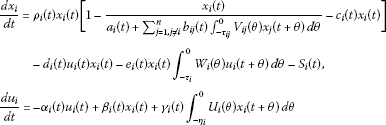admits at least one T-periodic solution. In order to indicate the validity of the assumptions made in our results, we also treat a illustrative example.

## References

1. May RM: Theoretical Ecology, Principles and Applications. Sounders, Philadelphia; 1976.

2. Cui JA, Chen LS: Global asymptotic stability in a nonautonomous cooperative system. Syst. Sci. Math. Sci. 1993, 6(1):44–51.

3. Cui JA: Global asymptotic stability in n -species cooperative system with time delays. Syst. Sci. Math. Sci. 1994, 7(1):45–48.

4. Zhang T, Li Y, Ye Y: Persistence and almost periodic solutions for a discrete fishing model with feedback control. Commun. Nonlinear Sci. Numer. Simul. 2011, 16(3):1564–1573. 10.1016/j.cnsns.2010.06.033

5. Yan J, Liu G: Periodicity and stability of a Lotka-Volterra competition system with feedback controls and deviating arguments. Nolinear Anal. 2011, 74: 2916–2928. 10.1016/j.na.2010.12.023

6. Chen F, Lio X, Huang X: The dynamic behavior of N -species cooperation system with continuous time delays and feedback controls. Appl. Math. Comput. 2006, 181: 803–815. 10.1016/j.amc.2006.02.007

7. Chen L, Xie X: Permanence of an N -species cooperation system with continuous time delays and feedback. Nonlinear Anal., Real World Appl. 2011, 12: 34–38. 10.1016/j.nonrwa.2010.05.033

8. Cauty R: Solution du problème de point fixe de Schauder. Fundam. Math. 2001, 170: 231–246. 10.4064/fm170-3-2

9. Dieudonné J: Foundations of Modern Analysis. Academic Press, San Diego; 1988.

10. Mokhtarzadeh MR, Pournaki MR, Razani A: A note on periodic solutions of Riccati equations. Nonlinear Dyn. 2010, 62: 119–125. 10.1007/s11071-010-9703-9

11. Chen F: On the periodic solutions of periodic multi-species Kolmogorov type competitive system with delays and feedback control. Appl. Math. Comput. 2006, 180: 366–373. 10.1016/j.amc.2005.12.014

12. Melnikov YA Topics in Engineering 27. In Green’s Functions in Applied Mechanics. Computational Mechanics, Southampton; 1995.

## Author information

Authors

### Corresponding author

Correspondence to Payam Nasertayoob.

## Rights and permissions

Reprints and Permissions

Nasertayoob, P., Vaezpour, S.M. Permanence and existence of positive periodic solution for a multi-species cooperation system with continuous time delays, feedback control, and periodic external source. Adv Differ Equ 2012, 156 (2012). https://doi.org/10.1186/1687-1847-2012-156

• Accepted:

• Published:

• DOI: https://doi.org/10.1186/1687-1847-2012-156

### Keywords

• Schauder’s fixed-point theorem
• periodic solution
• multi-species system
• feedback control